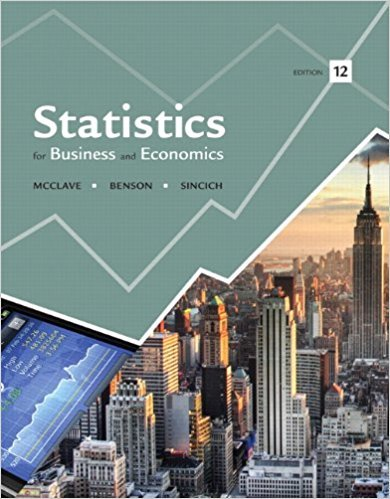×
×

# Investment risk analysis. The risk of a portfolio ofISBN: 9780321826237 51

## Solution for problem 32E Chapter 4

Statistics for Business and Economics | 12th Edition

• Textbook Solutions
• 2901 Step-by-step solutions solved by professors and subject experts
• Get 24/7 help from StudySoup virtual teaching assistantsStatistics for Business and Economics | 12th Edition

4 5 0 276 Reviews
18
4
Problem 32E

Investment risk analysis. The risk of a portfolio of financial assets is sometimes called investment risk. In general, investment risk is typically measured by computing the variance or standard deviation of the probability distribution that describes the decision maker’s potential outcomes (gains or losses). The greater the variation in potential outcomes, the greater the uncertainty faced by the decision maker; the smaller the variation in potential outcomes, the more predictable the decision maker’s gains or losses. The two discrete probability distributions given in the next table were developed from historical data. They describe the potential total physical damage losses next year to the fleets of delivery trucks of two different firms.

 Firm A Firm B Loss Next Year Probability Loss Next Year Probability \$ 0 .01 \$ 0 .00 500 .01 200 .01 1,000 .01 700 .02 1,500 0.02 1,200 0.02 2,000 .35 1,700 .15 2,500 .30 2,200 .30 3,000 .25 2,700 .30 3,500 .02 3,200 .15 4,000 .01 3,700 .02 4,500 .01 4,200 .02 5,000 .01 4,700 .01

a. Verify that both firms have the same expected total physical damage loss.

b. Compute the standard deviation of each probability distribution and determine which firm faces the greater risk of physical damage to its fleet next year.

Step-by-Step Solution:

Step 1 of 3:

Here the experiment under consideration is study of investment risk.

The data regarding the total physical damages losses next year for the fleets of the delivery trucks for the two firms is given.

This data is developed from the historical data.

 Firm A Firm B Loss next year Probability Loss next year Probability \$0 0.01 \$0 0 500 0.01 200 0.01 1,000 0.01 700 0.02 1,500 0.02 1,200 0.02 2,000 0.35 1,700 0.15 2,500 0.3 2,200 0.30 3,000 0.25 2,700 0.30 3,500 0.02 3,200 0.15 4,000 0.01 3,700 0.02 4,500 0.01 4,200, 0.02 5,000 0.01 4,700 0.01

Using this data we need to find the required values.

Step 2 of 3:

(a)

Here we need to find the expected total physical damage loss for the two firms and we have to check whether both the firms have same expected loss.

For the firm A, the  expected loss is given by

E(X)=xp(x)

=0(0.01)+500(0.01)+1000(0.01)+1500(0.02)+2000(0.35)+2500(0.3)+

3000(0.25)+3500(0.02)+4000(0.01)+4500(0.01)+5000(0.01)

=0+5+10+30+700+750+750+70+40+45+50

=2450

For the firm B,

E(X)=xp(x)

=0(0.00)+200(0.01)+700(0.02)+1200(0.02)+1700(0.15)+2200(0.30)+

2700(0.30)+3200(0.15)+3700(0.02)+4200(0.02)+4700(0.01)

=0+2+14+24+255+660+810+480+74+84+47

=2450

Thus, the two firms have the same expected physical damage loss.

Step 3 of 3

##### ISBN: 9780321826237

Unlock Textbook Solution